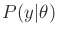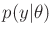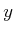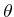### 9.5.2.3 Incorrect assumptions on conditional distributions

One final concern is that many times even the distributionis incorrectly estimated because it is assumed arbitrarily to belong to a family of distributions. For example, it is often very easy to work with Gaussian densities. Therefore, it is tempting to assume thatis Gaussian. Experiments can be performed to estimate the mean and variance parameters. Even though some best fit will be found, it does not necessarily imply that a Gaussian is a good representation. Conclusions based on this model may be incorrect, especially if the true distribution has a different shape, such as having a larger tail or being multimodal. In many cases, nonparametric methods may be needed to avoid such biases. Such methods do not assume a particular family of distributions. For example, imagine estimating a probability distribution by making a histogram that records the frequency ofoccurrences for a fixed value of. The histogram can then be normalized to contain a representation of the probability distribution without assuming an initial form.

Steven M LaValle 2020-08-14